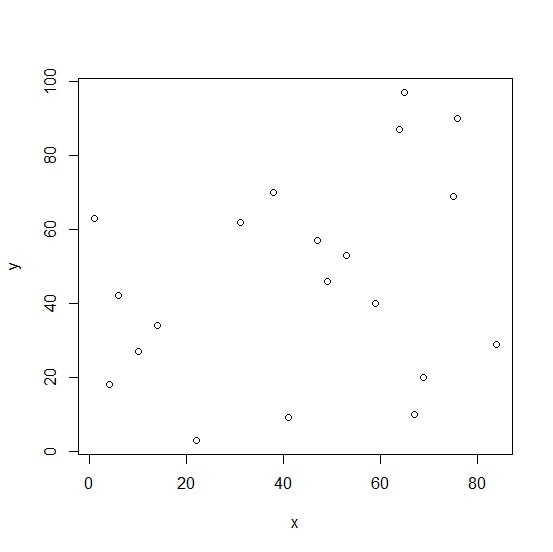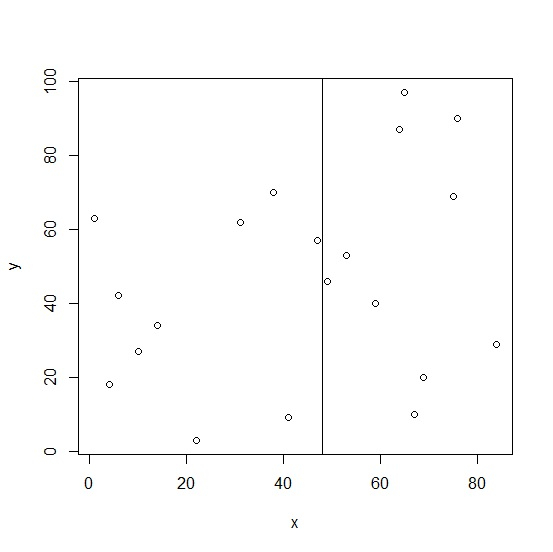# How to create vertical line for X variable at median in base R plot

To create vertical line for X variable at median in base R plot, we can follow the below steps −

• First of all, create two vectors and plot them.
• Create the vertical line at median using abline function.

## Create the vectors and plot them

Let’s create two random vectors and plot them as shown below −

### Example

Live Demo

x<-sample(1:100,20)
y<-sample(1:100,20)
plot(x,y)

On executing, the above script generates the below output(this output will vary on your system due to randomization) −

### Output## Create the vertical line at median

Use abline function with v argument to create the vertical line at median as shown below −

### Example

Live Demo

x<-sample(1:100,20)
y<-sample(1:100,20)
plot(x,y)
abline(v=median(x))

### Output